# Electronics and Communication Engineering - Exam Questions Papers

36.

A system with transfer function H(z) has impulse response h(.) defined as h(2) = 1, h(3) = -1 and h(k) = 0 otherwise. Consider the following statements
S1: H(z) is a low pass filter
S2: H(z) is a FIR filter.
Which of the following is correct?

 A. Only S2 is true B. Both S1 and S2 are false C. Both S1 and S2 are true, and S2 is a reason for S1 D. Both S1 and S2 are true, but S2 is not a reason for S1

Explanation:

H(e) = e-j2ω - e-j3ω. It is FIR high pass filter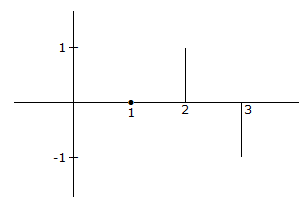37.

Consider an incremental vector element of length ΔL and angle between an electric field E and ΔL s cos θ.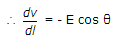Then in which direction should ΔL be placed to be obtain maximum value of Δv?

 A. In the direction of E B. In opposite direction of E C. In the angle of 45° with E D. In the angle of 60° with E

Explanation:

Now E is a definite value at the point which it is present and is independent of direction of ΔL.

The magnitude of ΔL is constant and direction is along the unit vector.

Maximum value of Δv will occur at cos θ = - 1 or ΔL points in opposite direction to E.

38.

A MOD - 2 counter, the FF has a tpd = 60 nsec. The NOR gate has a tpd of 30 nsec. The clock frequency is

 A. 37 MHz B. 40 MHz C. 111 MHz D. none

Explanation:

Clock period should be greater than or equal tpd

MOD-12 → X FF = 4 x 60 = 240 n sec

tpd of NOR gate = 30 n sec Total = 240 + 30 ⇒ 270 n sec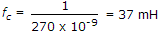.

39.

Evaluate the power stored in inductor and capacitor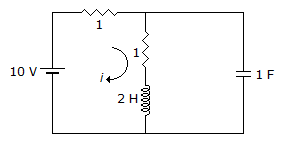A. 25 J, 12.5 J B. 12.5 J, 25 J C. 25 J, 50 J D. 12.5 J, 50 J

Explanation:

Energy stored in inductor L is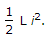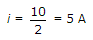as inductor will act as a short circuit for dc.

EL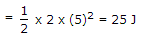Energy stored in capacitor C is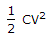Voltage across capacitor C is 5 V (i.e. voltage across shunt resistor R)

EC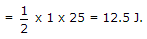40.

If the Laplace transform of the voltage transform of the voltage across a capacitor of value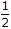F is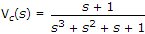the value of the current through the capacitor at t = 0+ is

 A. 0 A B. 2 A C.A D. 1 A

Explanation: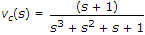capacitor =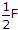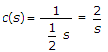I(s) = vc(s)/c(s)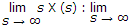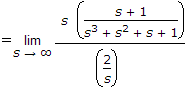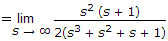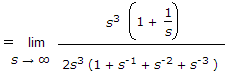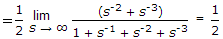.

#### Current Affairs 2022

Interview Questions and Answers